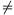Mathematical and Physical Journal
for High Schools
Issued by the MATFUND Foundation
 Already signed up? New to KöMaL?

#Problem A. 432. (September 2007)

A. 432. Find all integer a for which there exists distinct positive integers x and y such that (axy+1) divides (ax2+1)2.

(5 pont)

Deadline expired on October 15, 2007.

Solution. We show that the desired positive integers x,y exist if and only if a-1.

For a=-1, a suitable pair is x=1, y=2 since (ax2+1)2=0.

For a0, the pair x=1, y=a+2 is suitable since x<y and (ax2+1)2=axy+1=(a+1)2.

Now consider the case a<-1. Let b=|a|2. Call a pair (x,y) of positive integers bad, if xy and |axy+1|=(bxy-1) divides (ax2+1)2=(bx2-1)2. To the contrary, suppose that there exist at least one bad pair and consider that pair where x is minimal; if there are more than one such pair, then take the smallest possible y for that value of x.

Case 1: x>y. Sinceand(by2-1)2 is also divisible by (bxy-1). So the pair (y,x) is bad. But y<x which contradicts that we chose the smallest possible x.

Case 2: x<y. Consider the number. Both the numerator and the denominator are positive because b2 and x,y1; therefore c is a positive integer. Considering modulo bx,hence c=bxz-1 with some positive integer z; then

(bx2-1)2=(bxy-1)(bxz-1).

From x<y we obtain z<x. Then the pair (x,z) is bad. Since z<x<y, this contradicts the choice of y.

Both cases lead to contradiction; bad pairs do not exist for b2.

### Statistics:

 11 students sent a solution. 5 points: Korándi Dániel, Lovász László Miklós, Nagy 235 János, Nagy 314 Dániel, Tomon István, Wolosz János. 4 points: Huszár Kristóf. 1 point: 1 student. 0 point: 2 students. Unfair, not evaluated: 1 solutions.

Problems in Mathematics of KöMaL, September 2007